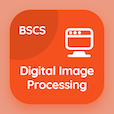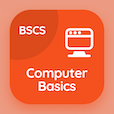Computer Science Courses

Digital Image Processing Quiz Questions

Digital Image Processing Practice Test 40

# Introduction to Wavelet and Multiresolution Processing Test Questions PDF - 40

The Book Introduction to Wavelet and Multiresolution Processing Test Questions PDF, introduction to wavelet and multiresolution processing quiz answers PDF, download chapter 10-40 to learn online digital image processing course. Solve Wavelet and Multiresolution Processing Test PDF, introduction to wavelet and multiresolution processing Multiple Choice Questions (MCQ Quiz) for online college degrees. The eBook Introduction to Wavelet and Multiresolution Processing Trivia App Download: introduction to wavelet and multiresolution processing, point line and edge detection in image processing, preview in image segmentation, edge detection in image processing, morphological opening closing test prep to learn online certificate courses.

The Test: Modulated version of filter is defined by the equation PDF, "Introduction to Wavelet and Multiresolution Processing Test" App APK Download with h5(n) = h1(n), h5(n) = (-1)nh1(n), h5(n) = (-1)n, and h = (-1)nh1(n) choices for computer information science. Study wavelet and multiresolution processing questions and answers, Apple Book to download free sample for computer software engineer online degree.

## Image Processing: Introduction to Wavelet & Multiresolution Processing MCQs Quiz

MCQ: Modulated version of filter is defined by the equation

A) h5(n) = (-1)nh1(n)
B) h5(n) = h1(n)
C) h5(n) = (-1)n
D) h = (-1)nh1(n)

MCQ: First derivative approximation says that values of intensities at the onset must be

A) nonzero
B) zero
C) positive
D) negative

MCQ: Image segmentation is also based on

A) morphology
B) set theory
C) extraction
D) recognition

MCQ: Image whose principle features are edges is called

A) orthogonal
B) isolated
C) edge map
D) edge normal

MCQ: Opening and closing are each others

A) neighbors
B) duals
C) centers
D) corners

### Mock Tests: Digital Image Processing Course Prep

Download Digital Image Processing Quiz App, DBMS MCQ App, and Computer Basics MCQs App to install for Android & iOS devices. These Apps include complete analytics of real time attempts with interactive assessments. Download Play Store & App Store Apps & Enjoy 100% functionality with subscriptions!